﻿ 一种高效的稀疏卷积神经网络加速器的设计与实现
«上一篇文章快速检索 高级检索

 智能系统学报2020, Vol. 15Issue (2): 323-333  DOI: 10.11992/tis.2019020070

### 引用本文YU Chengyu, LI Zhiyuan, MAO Wenyu, et al. Design and implementation of an efficient accelerator for sparse convolutional neural network[J]. CAAI Transactions on Intelligent Systems, 2020, 15(2): 323-333. DOI: 10.11992/tis.201902007.### 文章历史

1. 中国科学院 半导体研究所，北京 100083;
2. 中国科学院大学，北京 100089;
3. 中国科学院 脑科学与智能技术卓越创新中心，上海 200031;
4. 半导体神经网络智能感知与计算技术北京市重点实验室，北京 100083

Design and implementation of an efficient accelerator for sparse convolutional neural network
YU Chengyu 1,2, LI Zhiyuan 1,2, MAO Wenyu 1, LU Huaxiang 1,2,3,4
1. Institute of Semiconductors, Chinese Academy of Sciences, Beijing 100083, China;
2. University of Chinese Academy of Sciences, Beijing 100089, China;
3. Center for Excellence in Brain Science and Intelligence Technology, Chinese Academy of Sciences, Shanghai 200031, China;
4. Semiconductor Neural Network Intelligent Perception and Computing Technology Beijing Key Lab, Beijing 100083, China
Abstract: To address the difficulty experienced by convolutional neural networks (CNNs) in computing hardware implementation, most previous designs of convolutional neural network accelerators have focused on solving the computation performance and bandwidth bottlenecks, while ignoring the importance of CNN sparsity to accelerator design. Recently, it has often been difficult to simultaneously achieve computational flexibility, parallel efficiency, and resource overhead using the small number of CNN accelerator designs capable of utilizing sparsity. In this paper, we first analyze the effects of different parallel expansion methods on the use of sparsity, analyze different methods that utilize sparsity, and then propose a parallel expansion method that can accelerate CNNs with activated sparsity to achieve higher parallelism efficiency and lower additional resource cost, as compared with other designs. Lastly, we complete the design of this CNN accelerator and implemented it on FPGA. The results show that compared with a dense network design using the same device, the acceleration performance of the VGG-16 network was increased by 108.8% and its overall performance was improved by 164.6%, which has obvious performance advantages.
Key words: convolutional neural network    sparsity    embedded FPGA    ReLU    hardware acceleration    parallel computing    deep learning

1 卷积神经网络的稀疏性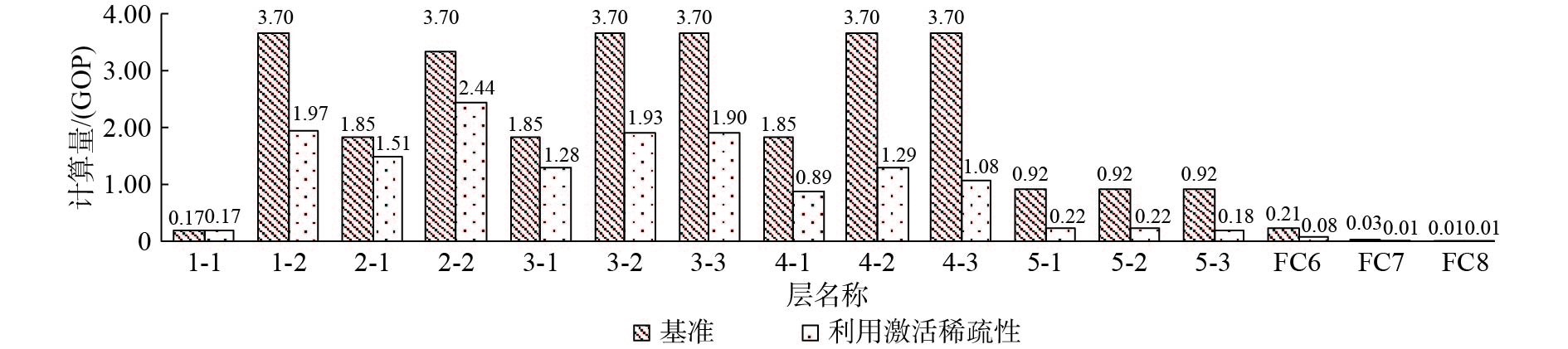Download: 图 1 利用激活稀疏性前后VGG-16网络每层计算量的变化 Fig. 1 Changes of the computation amount of VGG-16 network layer before and after utilizing the sparsity of activation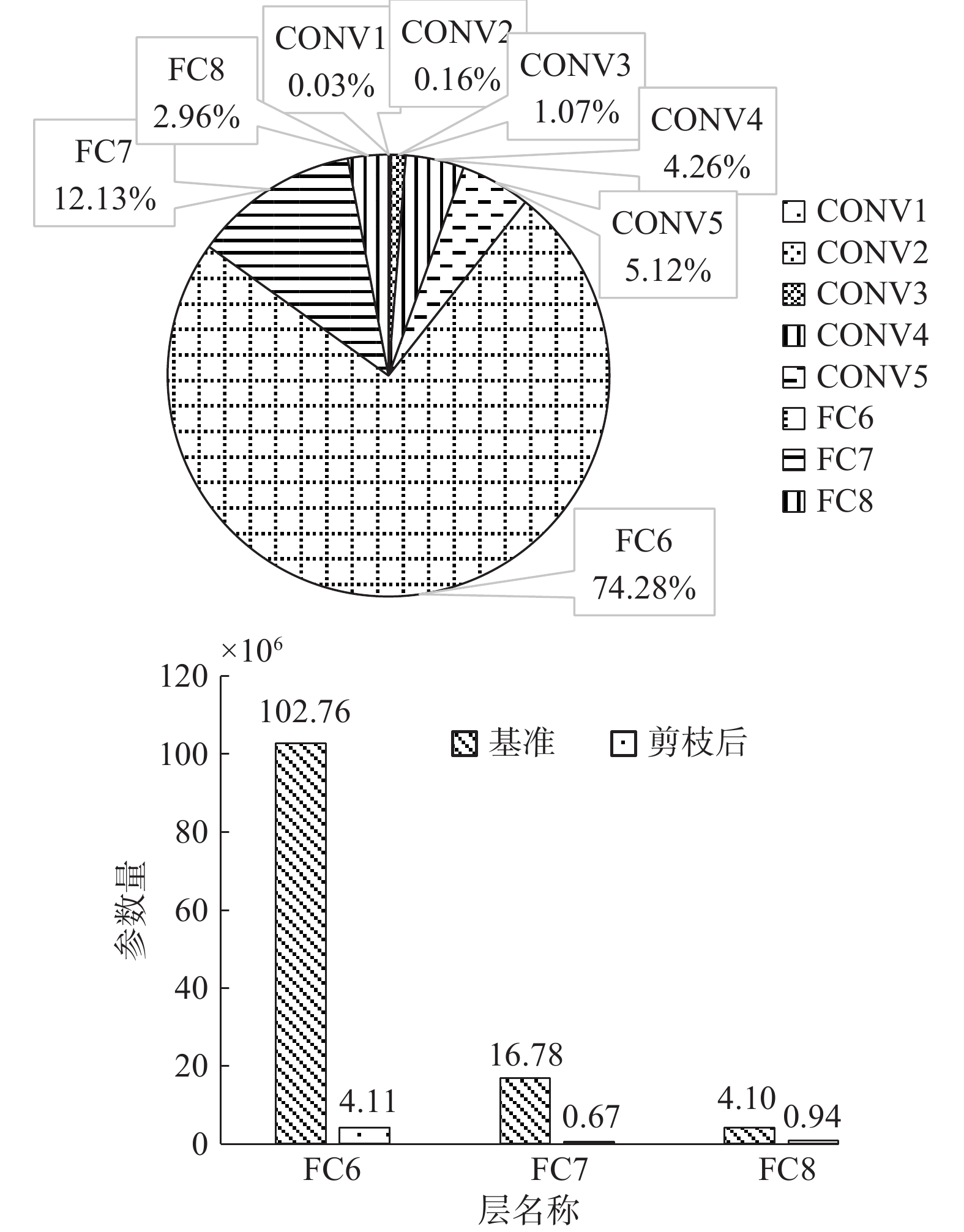Download: 图 2 VGG-16全连接层参数量比重与剪枝前后的变化 Fig. 2 Proportion and Changes of the parameters amount of VGG-16 full connected layer before and after pruning
2 算法理论设计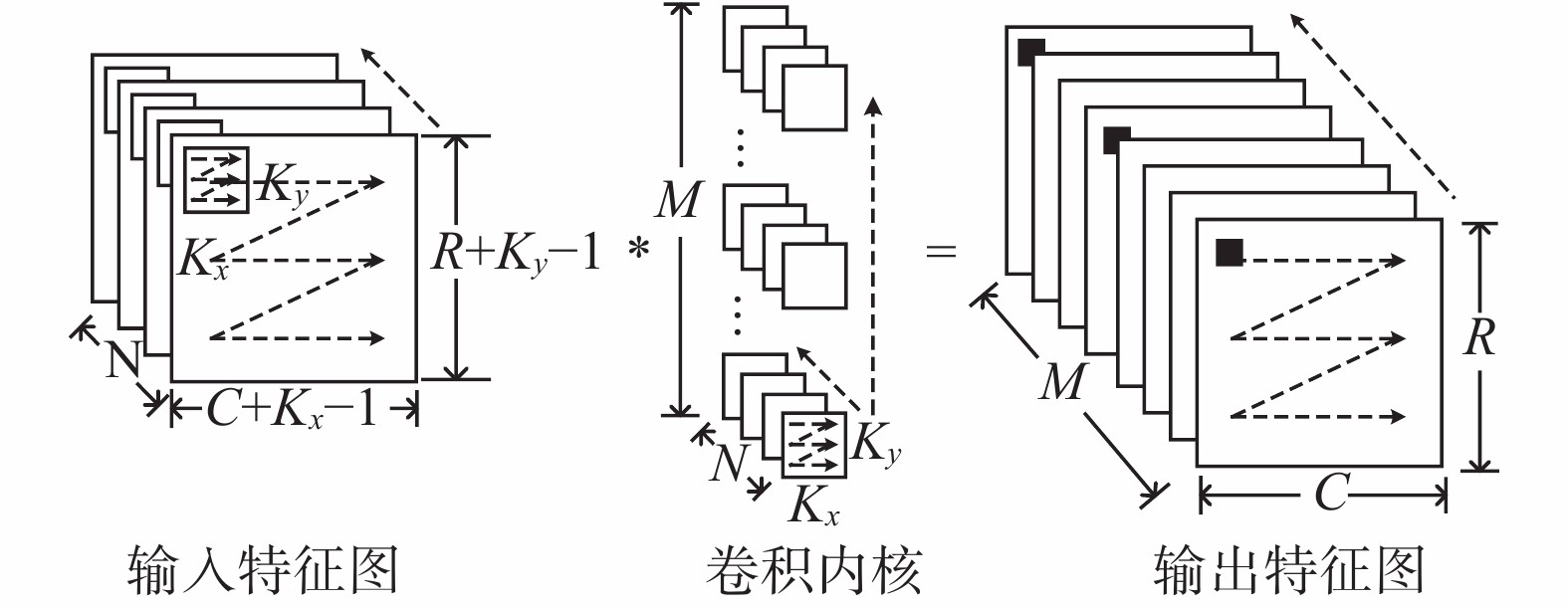Download: 图 3 卷积层计算示意 Fig. 3 Schematic diagram of convolution computing
 算法1　典型的卷积计算伪代码 ${\rm{for}}\left( {m = 0;m < M;m + + } \right)\{$ //输出特征图 ${\rm{for}}\left( {n = 0;n 利用稀疏性加速卷积神经网络计算会给硬件上带来两个问题：首先，稀疏的卷积计算是不平衡的，而并行计算时负载失衡会导致计算单元利用率下降，损失性能，在并行队列数量较多、队列长度不够长时，负载失衡的影响会非常明显；其次，对激活输入和权重的判断或选择会增加逻辑复杂度，可能会带来不能接受的面积开销，影响并行展开规模。 用系统并行自由度来表征并行计算的负载失衡。如图4所示，大部分卷积计算是以窗口滑动为时间基本单位控制时序的，窗口滑动之间需要等待所有并行化方向上的计算队列计算完成，所以系统并行自由度越高，负载失衡造成的性能损失就越严重。对于完全规则设计的卷积计算模型，所有并行计算队列的计算时间是相同的，这样的系统的并行自由度为1，而增加对权重$K_{\left( {i,j} \right)}^{\left( {m,n} \right)}$和对激活输入$I_{\left( {c + i,r + j} \right)}^{\left( n \right)}$的选择会使子队列长度不均等，增加系统并行自由度。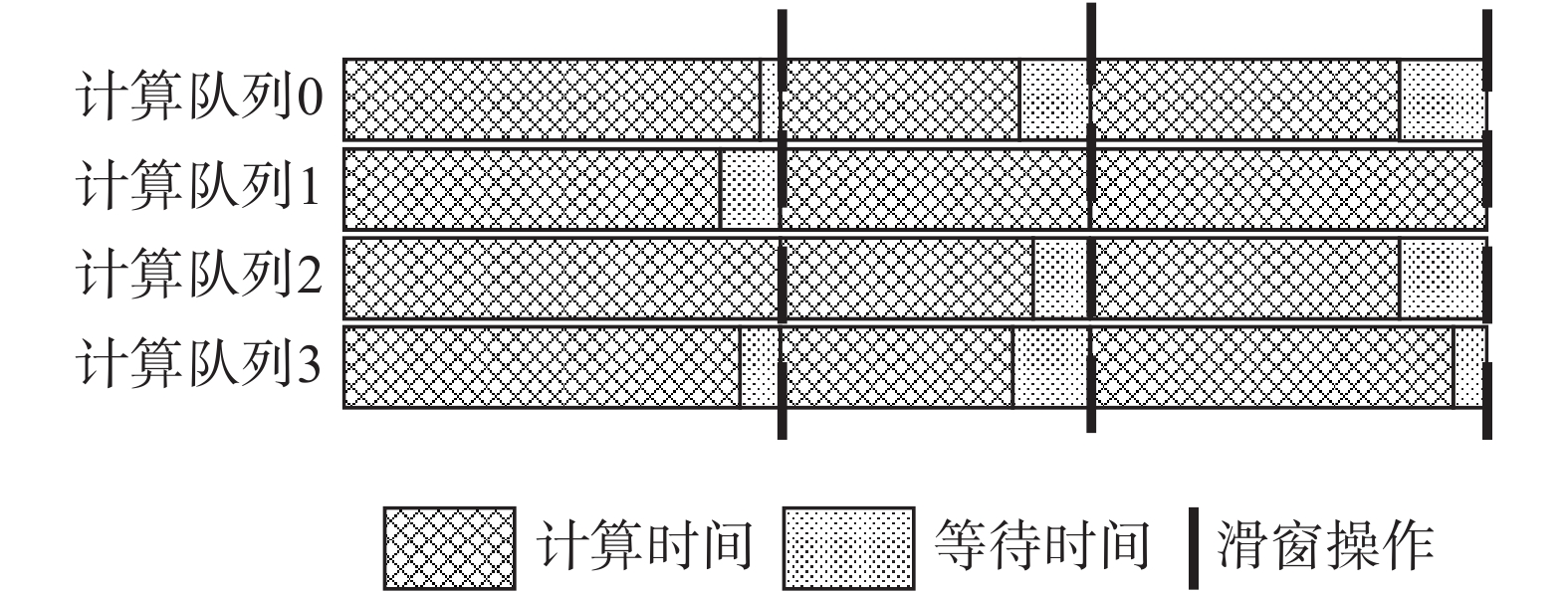Download: 图 4 并行计算性能损失示意 Fig. 4 Schematic diagram of parallel computing performance losses 卷积计算硬件化设计的关键在于如何兼顾数据共享、复用和计算的灵活性，对于稀疏的卷积计算，需要最大化数据共享来降低并行计算时负载失衡导致的性能损失。本文分别从并行展开方式和利用稀疏性方式进行分析比较，确定并行效率极高、额外资源开销较小的稀疏卷积神经网络加速器设计。 2.1 并行展开方式 图5所示，总体来说，卷积神经网络加速器设计的卷积计算的并行展开方式可以分为3种：展开突触(KxKy)、展开神经元(RC)、展开特征图(MN)。大部分卷积神经网络加速器的设计都是按照这3种并行展开方式的其中的一种或者多种展开的 对突触进行并行展开通常使用脉动阵列的方式。如图5(a)所示，图中Kx=Ky=3，PE缓存权重，按照内核行方向Kx从左到右级联，每次计算时PE将乘法运算结果与左边相邻PE输入的值做加法运算送往右边相邻PE，每行最右边PE通过一个深度为R的FIFO连接到次行，脉动阵列的输入为0，通过全部9个PE之后计算完成全部内核方向的计算。这种并行展开方式共享了激活输入，复用了权重。它利用激活稀疏性的系统并行自由度为1，利用参数稀疏性的系统并行自由度为Kx×Ky，同时利用两种稀疏性的系统并行自由度为Kx×Ky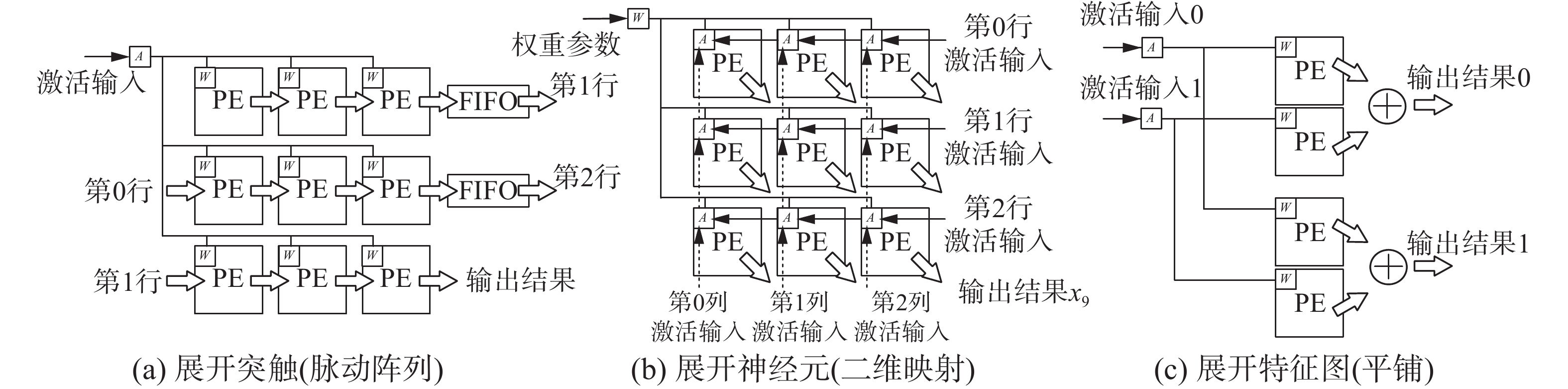Download: 图 5 并行展开方式示意 Fig. 5 Schematic diagram of parallel expansion mode 对神经元进行并行展开通常使用二维映射的方式，如图5(b)所示，特征图的行方向R和列方向C被分块为TrTc，图中Tr=Tc=3，计算开始时，Tr×Tc的激活输入被初始化到Tr×Tc个PE上，权重$K_{\left( {i,j} \right)}^{\left( {m,n} \right)}$广播到每个PE完成乘累加计算，每个PE分别缓存计算结果，每次计算时PE向左或者向上将激活输入传递给相邻PE，依次按照内核行方向Kx和列方向Ky输入权重并传递激活输入，经过Kx×Ky个周期，计算完成，每个PE完成了对应神经元的全部内核方向的乘累加计算。这种并行展开方式共享了权重，复用了激活输入。它利用激活稀疏性的系统并行自由度为Tr×Tc，利用参数稀疏性的系统并行自由度为1，同时利用两种稀疏性的系统并行自由度为Tr×Tc 对特征图进行并行展开通常使用平铺的方式。如图5(c)所示，输入特征图N和输出特征图M被分块为TnTm图5Tn=1，Tm=2，对应相同输入特征图的乘法器共享激活输入，对应相同输出特征图的乘法器将计算结果经由一个加法树累加输出。这种并行展开方式共享了激活输入。它利用激活稀疏性的系统并行自由度为Tn，利用参数稀疏性的系统并行自由度为Tm，同时利用两种稀疏性的系统并行自由度为Tn×Tm 假设同时利用权重$K_{\left( {i,j} \right)}^{\left( {m,n} \right)}$和激活输入$I_{\left( {c + i,r + j} \right)}^{\left( n \right)}$的稀疏性，由上文的分析可以看出，无论从什么方向对计算进行并行化，都会增加系统并行自由度，系统并行自由度等于PE展开数量。可以预见在一个没有经过特殊均衡化处理的网络上，由于负载失衡造成的性能损失将是非常严重的。与此同时，只要利用参数稀疏性，这3种并行展开方式目前的数据共享与复用都会遭到不同程度的破坏，每个PE都需要独立的选择与索引逻辑，这导致单位PE面积可能会非常巨大，不利于大规模并行。尤其是目前看来，对于计算量密集的卷积计算，通过利用参数稀疏性节省的计算量可能还不能抵消资源上的开销，这是得不偿失的。 基于以上这些考虑，在卷积层计算时仅利用了激活输入$I_{\left( {c + i,r + j} \right)}^{\left( n \right)}$的稀疏性。如图6所示，首先使用平铺的方式对输出特征图方向部分并行展开了Tm长度，这Tm个PE组成一个共享激活输入的PE组，然后将Kx×Ky个这样的PE组按突触方向使用脉动阵列的方式并行展开。使用这样的并行展开方式，展开了Tm×Kx×Ky个PE，而整个系统仅使用了1个逻辑单元负责有效激活输入选择，就完成了利用激活稀疏性加速计算的功能，资源开销极小，并且系统并行自由度仅为1,负载失衡造成的性能损失最低。对应的卷积层计算伪代码如算法2所示。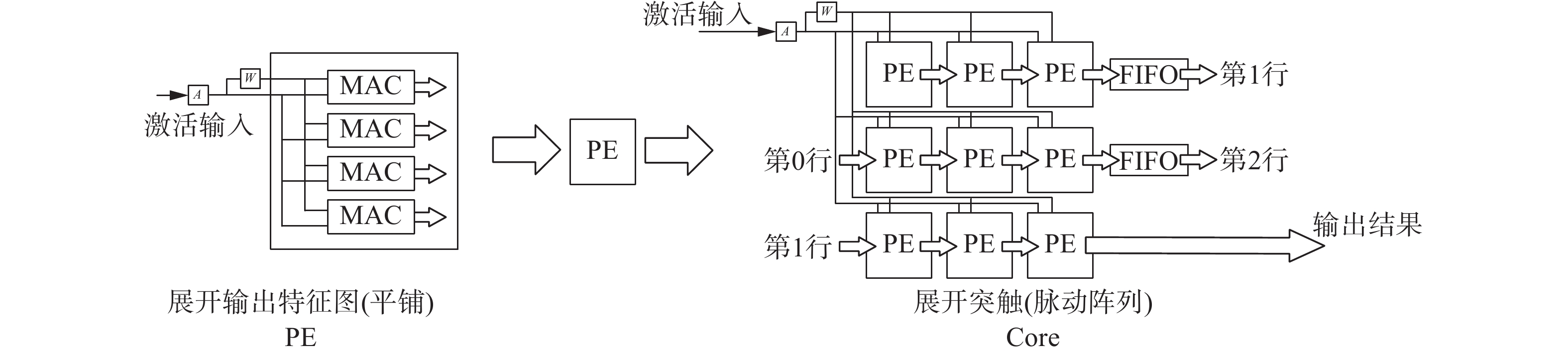Download: 图 6 本设计使用的并行展开方式示意 Fig. 6 Schematic diagram of parallel expansion mode in our design  算法2 卷积层计算伪代码${\rm{for} }\left( {m = 0;m < M;m + = T_m} \right)\{ K = {\rm{load}}\_{\rm{weight}}({{m);}}{\rm{for}}\left( {c = 0;c < C;c + + } \right)\{ {\rm{for}}\left( {r = 0;r < R;r + + } \right)\{ {\rm{for}}\left( {n = 0;n < N;n + + } \right)\{ {\rm{if}}\left( {{\rm{}}I_{\left( {r,c + j} \right)}^{\left( {tn} \right)} \ne 0{\rm{}}} \right)\{ {\rm{for} }\left( {j = 0;j < K_y;j + + } \right)\{ {\rm{}}\# {\rm{unroll}}{\rm{for} }\left( {i = 0;i < K_x;i + + } \right)\{ )\{ {\rm{}}\# {\rm{unroll}}{\rm{for} }\left( {{\rm tm} = m; {\rm tm} < \min \left( {m + {T_m},M} \right);tm + + } \right)\{ {\rm{}}\# {\rm{unroll}} S_{\left( {c,r} \right)}^{\left( {tm} \right)}\left[ i \right]\left[ j \right] + = K_{\left( {i,j} \right)}^{\left( {tm,n} \right)} \times I_{\left( {c,r} \right)}^{\left( n \right)};{\rm{}}\} {\rm{}}\} {\rm{}}\} {\rm{}}\} {\rm{}}\} {\rm{}}\} O_{\left( {c,r} \right)}^{\left( {tm} \right)} = {\rm Systolic}\left( {\displaystyle\sum \limits_{j = 0}^{Ky - 1} \displaystyle \sum \limits_{i = 0}^{Kx - 1} S_{\left( {c + i - Kx,r + j - Ky} \right)}^{\left( {tm} \right)}\left[ i \right]\left[ j \right]} \right);\} {\rm{}}\} {\rm{}}\} $注意到现代卷积神经网络的卷积核几乎只有3×3和1×1，并且对于大于3×3的卷积核(例如5×5)可以使用多次3×3卷积替代。类似的，每个PE组分别独立计算输出特征图即可以将3×3卷积工作模式转换成1×1卷积工作模式。所以本文提出的这种并行展开方式展开3×3的卷积核是可以适应于大部分卷积神经网络的。 全连接层参数量巨大，片上片下传输带宽是全连接层计算瓶颈，不做任何算法优化在硬件上完成全连接层计算是效率极低的，并且本身全连接层稀疏性非常高(对于VGG-16，FC-6的有效参数密度为4%)，计算队列的长度特别长(VGG-16，不分块情况下FC-6的激活队列长度为25 088)，足以忽略负载失衡造成的性能损失，非常适合利用参数稀疏性进行计算，所以对全连接层计算使用压缩稀疏列(CSC)方式同时使用了激活稀疏性和参数稀疏性。全连接层计算是简单的矩阵乘法，使用平铺的方式并行展开输出特征图效率最高，此时系统并行自由度为Tm。对应的全连接层计算伪代码如算法3所示。  算法3 全连接层计算伪代码$ {\rm{for} }\left( {m = 0;m < M;m + = T_m} \right)\{  {\rm{for} }\left( {n = 0;n < N;n + = T_n} \right)\{  K = {\rm{load}}\_{\rm{weight}}({{m,n);}} {\rm{for} }\left( { {\rm tn} = n;{\rm tn} < {\rm min} \left( {n + T_n,N} \right);{\rm tn} + + } \right)\{  \# {\rm{pipeline}} {\rm{if}}\left( {{\rm{}}{I^{\left( {tn} \right)}} \ne 0{\rm{}}} \right)\{  {\rm{for} }\left( {{\rm tm} = m;{\rm tm} < {\rm min} \left( {m + T_m,M} \right);{\rm tm} + = {\rm Tmm}} \right)\{  \# {\rm{parallelism}} {\rm{for} }\left( {{\rm tmm} = {\rm tm};{\rm tmm} < {\rm min} \left( {{\rm tm} + {\rm Tmm},M} \right);{\rm tmm} + + } \right)\{  \# {\rm{pipeline}} {\rm{if} }\left( { {\rm{} }{K^{\left( {\rm tmm,tn} \right)} } \ne 0{\rm{} } } \right)\{  {O^{\left( {\rm tmm} \right)} } + = {K^{\left( {\rm tmm,tn} \right)} } \times {I^{\left( {\rm tn} \right)} }; \} {\rm{}}\} {\rm{}}\} {\rm{}}\} {\rm{}}\} {\rm{}}\} {\rm{}}\} \$
2.2 利用稀疏性方式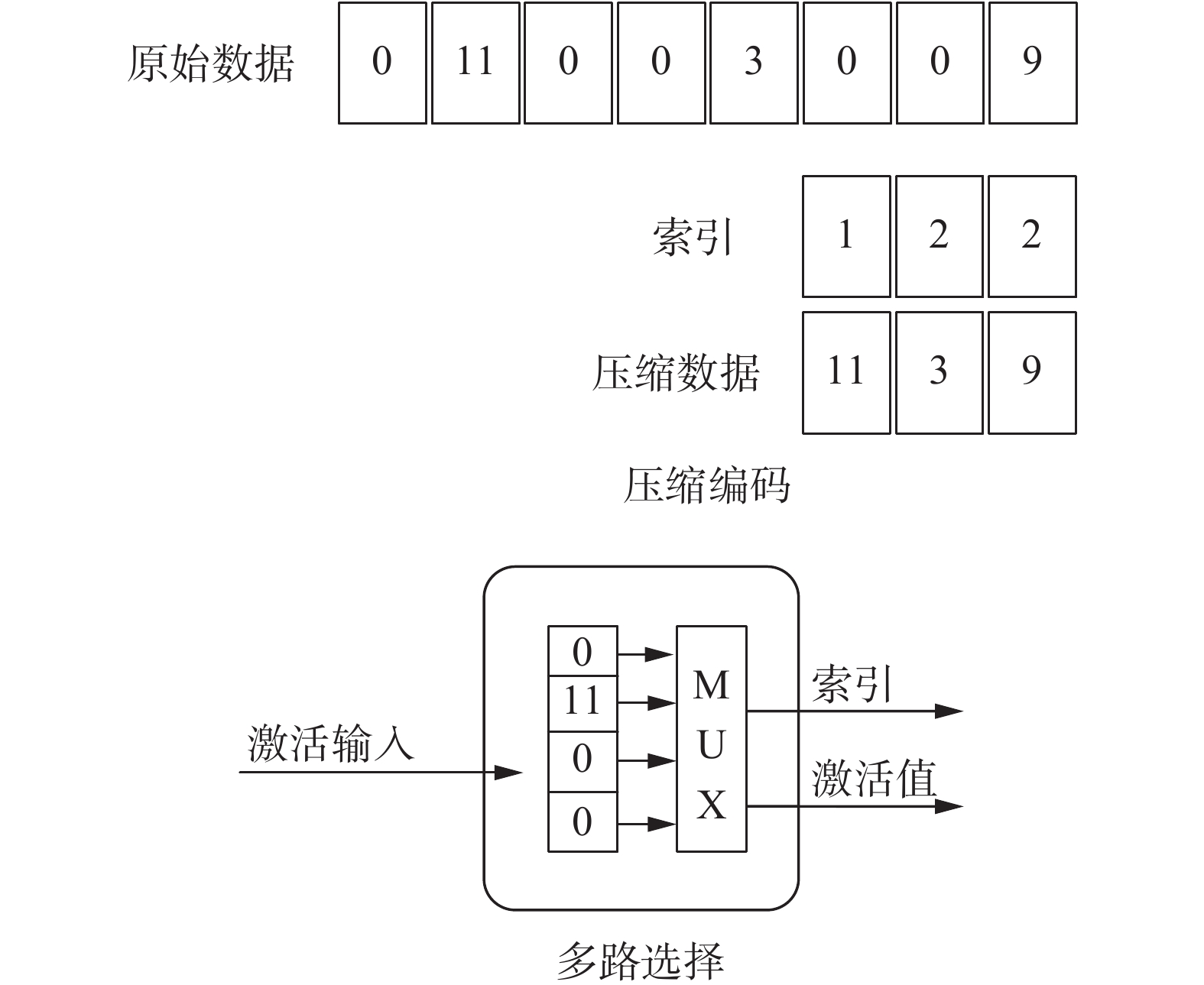Download: 图 7 利用激活稀疏性的方式 Fig. 7 Method of using activate sparsity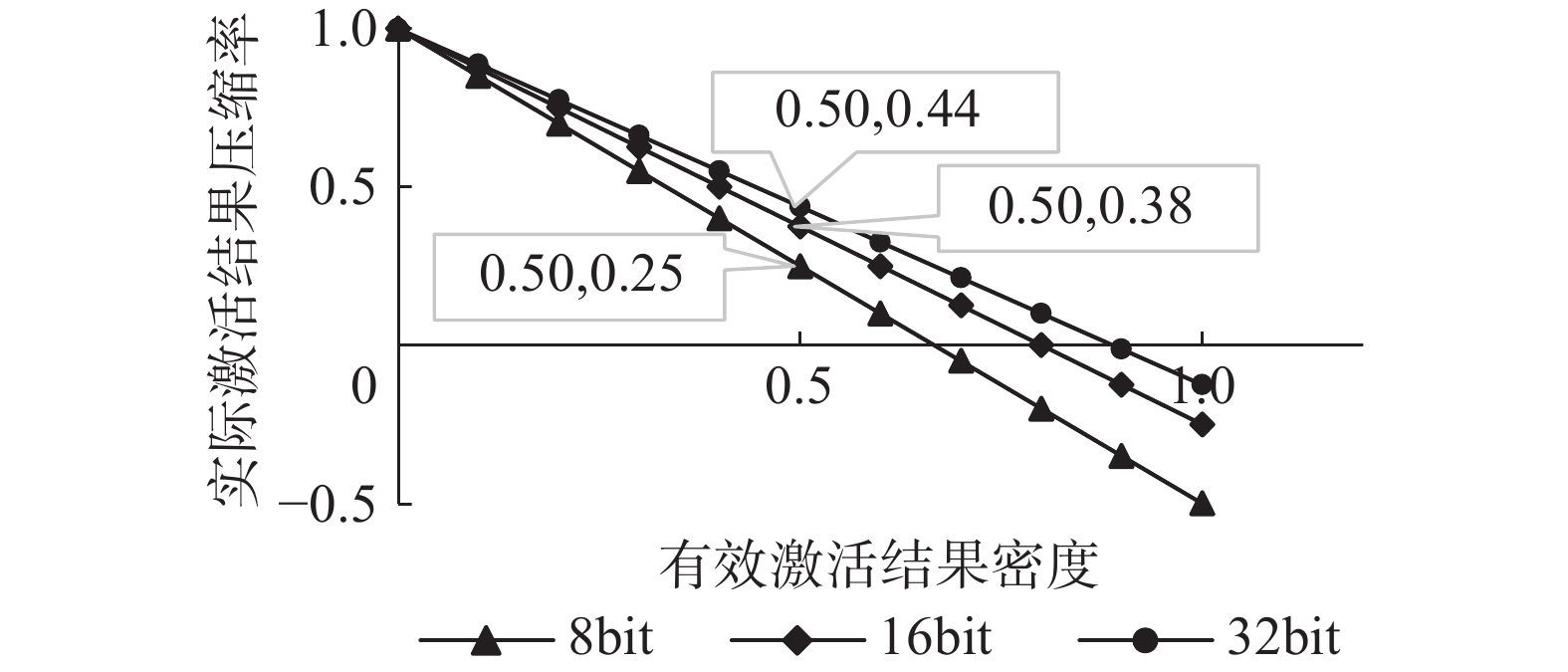Download: 图 8 不同量化精度下VGG-16压缩编码实际压缩效率 Fig. 8 Actual compression efficiency of VGG-16 compression code with different quantization precision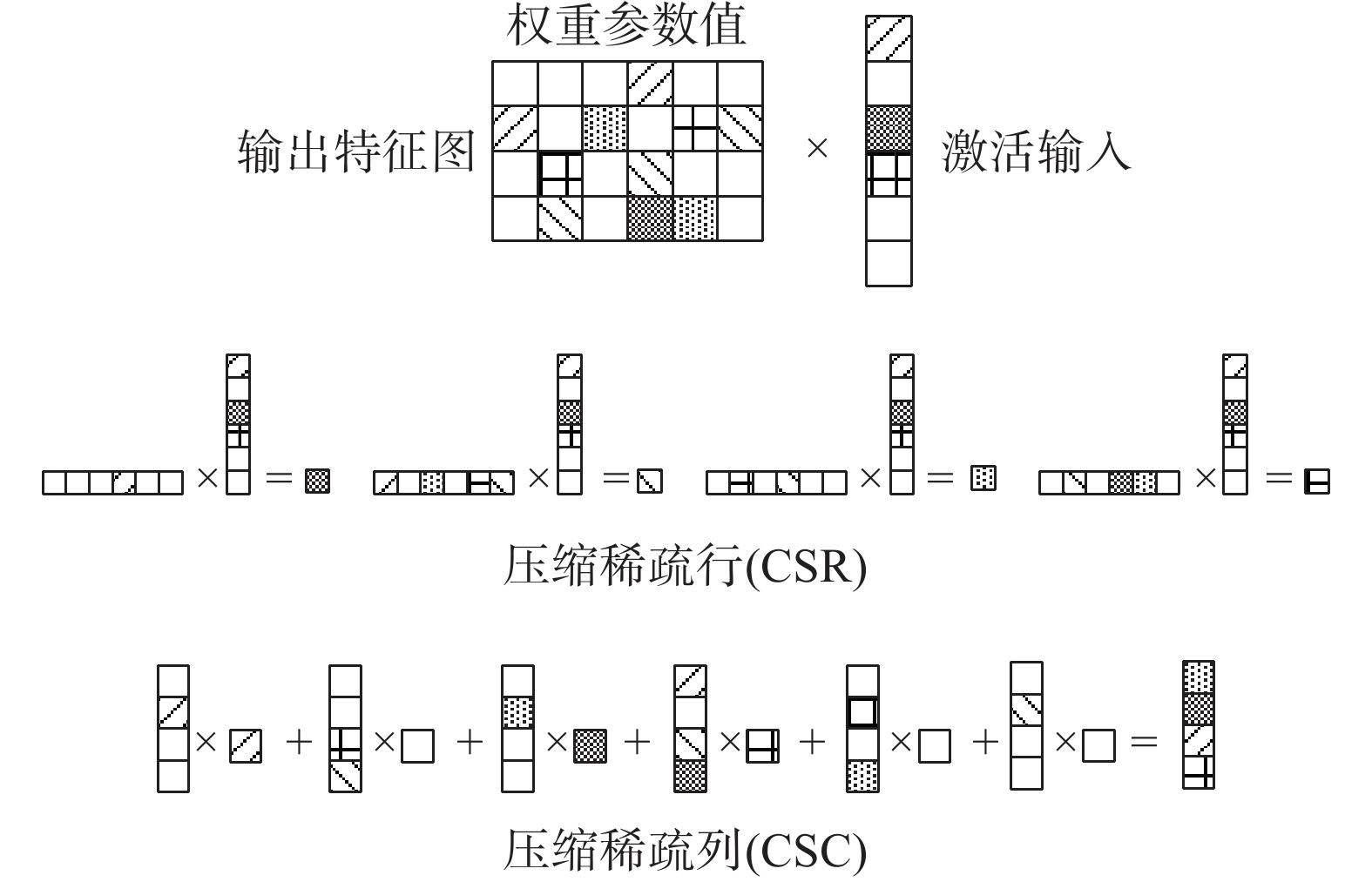Download: 图 9 利用参数稀疏性方式 Fig. 9 Method of using parameter sparsity

CSR模式需要完成两个稀疏矩阵的乘法，复杂性较高，文献利用笛卡尔乘法在CSR模式完成了稀疏矩阵计算，文献使用CSR模式仅利用了参数稀疏性。CSC模式将计算分离成了若干个计算队列，选择有效队列就能利用激活稀疏性，更容易实现，文献使用了CSC模式同时利用了激活稀疏性和参数稀疏性。使用CSC模式完成全连接层计算。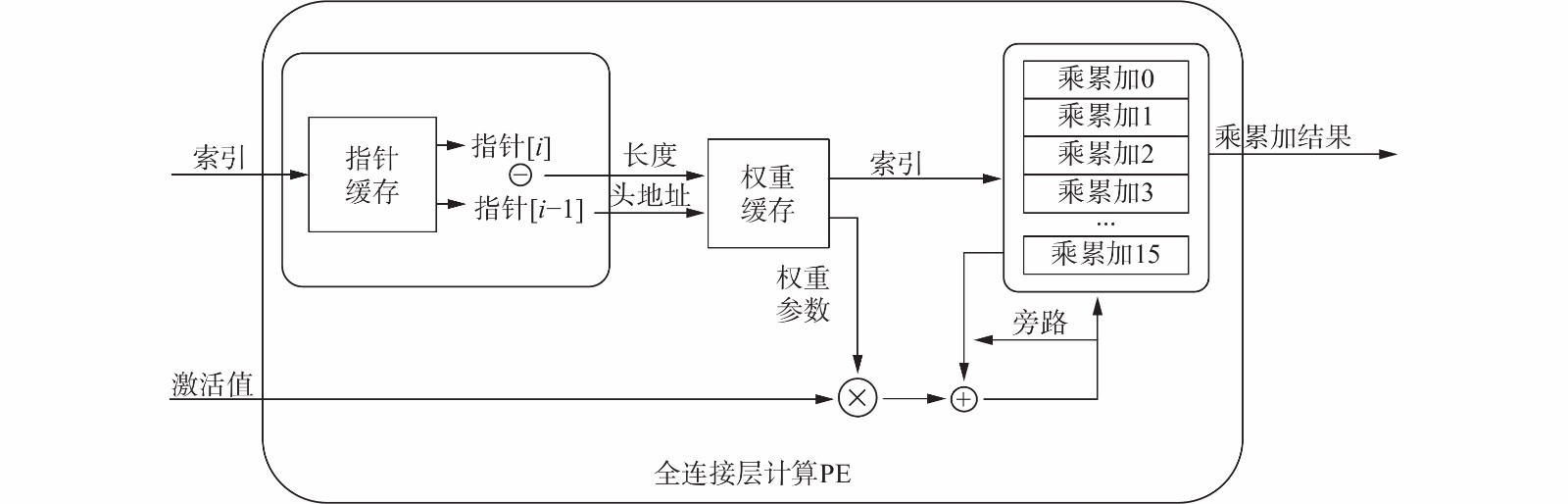Download: 图 10 全连接层稀疏计算PE示意 Fig. 10 Schematic diagram of sparse computing PE for FC layer
2.3 与其他加速器设计的比较表 1 并行展开方式和利用稀疏性的方式比较 Tab.1 Compare of parallel expansion and method of using sparsity

3 硬件设计与实现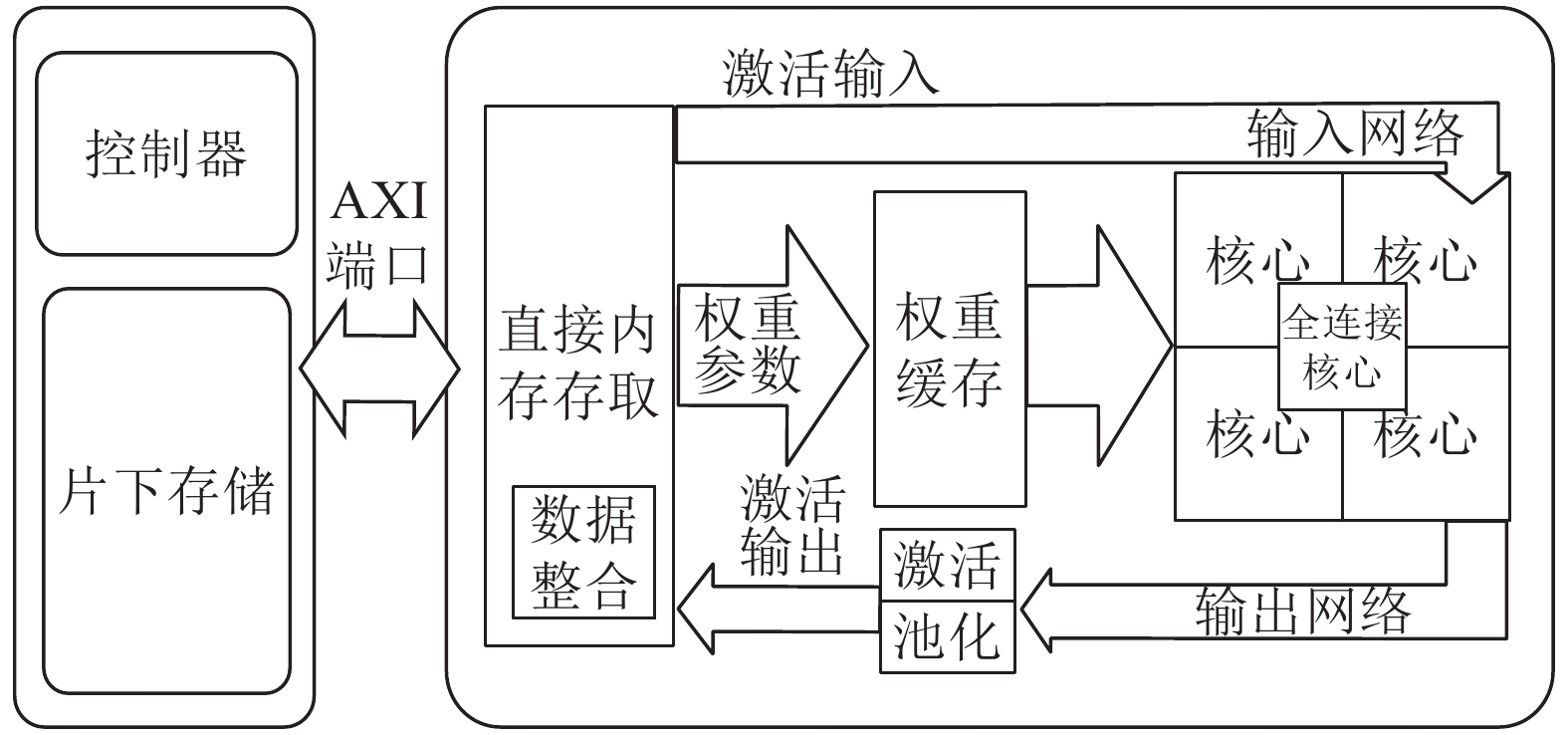Download: 图 11 系统整体架构 Fig. 11 Schematic diagram of the overall architecture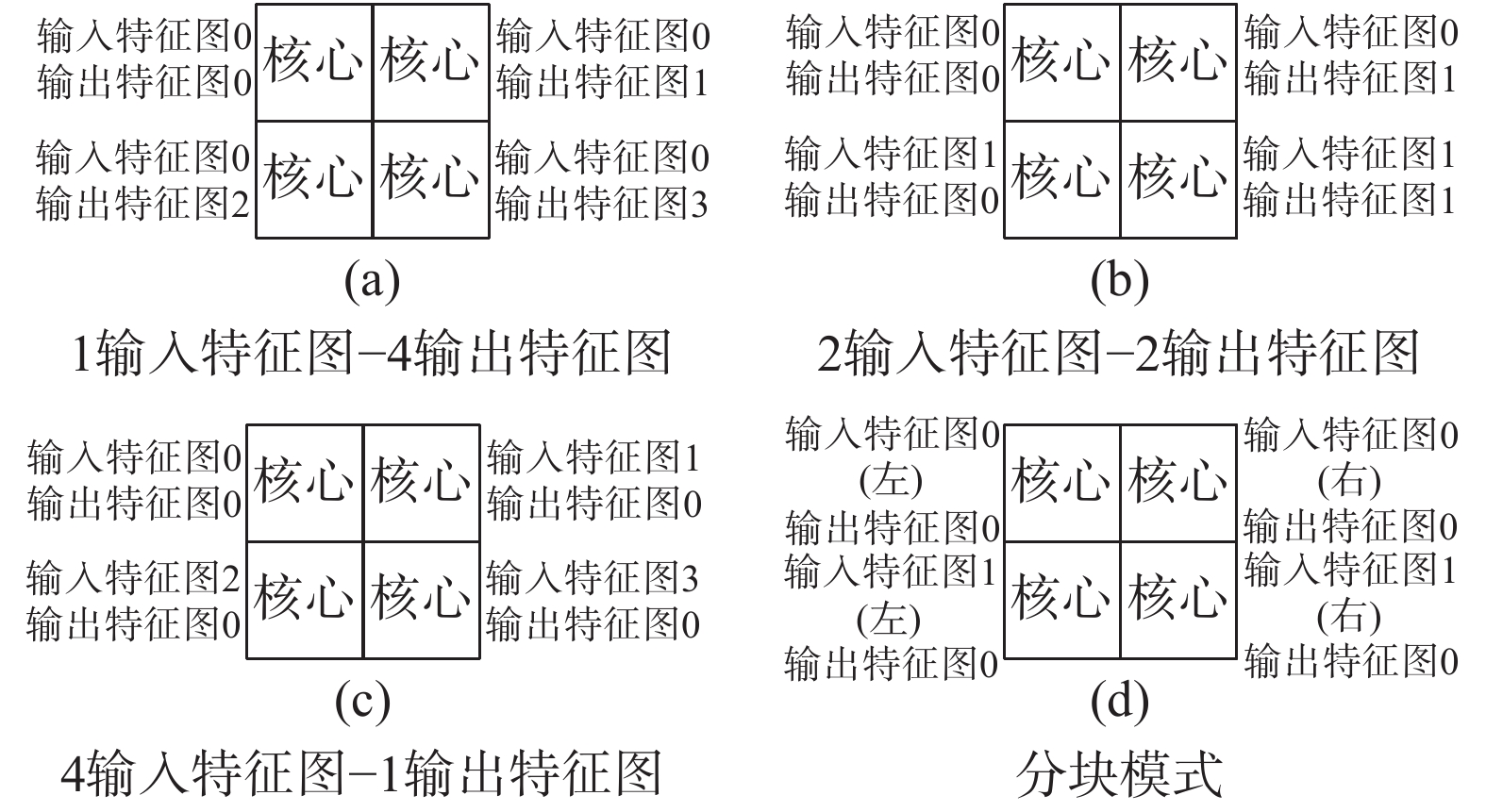Download: 图 12 多种工作模式示意 Fig. 12 Schematic diagram of multiple working modes表 2 FPGA资源使用情况 Tab.2 Utilization report of FPGA

4 性能分析评估与比较 4.1 加速效果与并行展开方式比较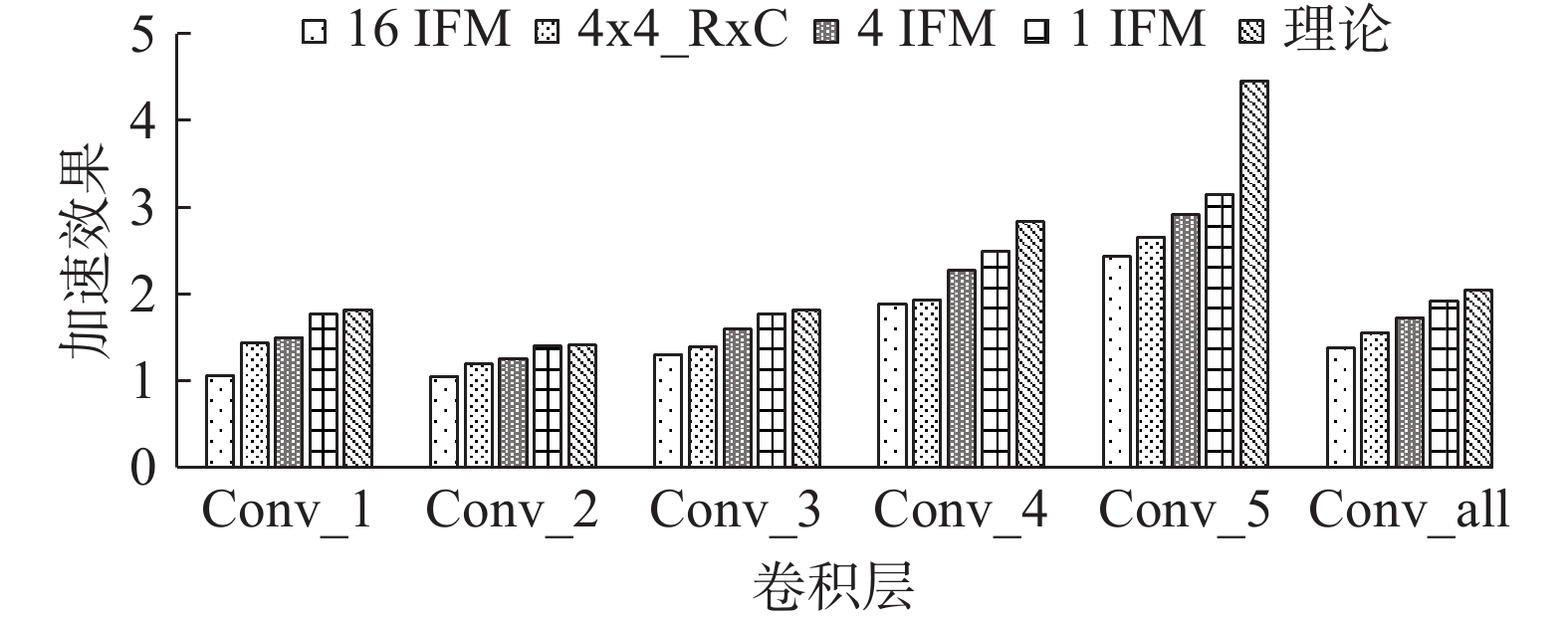Download: 图 13 VGG-16网络不同的并行展开方式加速效果比较 Fig. 13 Comparison of acceleration effects of VGG-16 networks with different parallel expansion modes
4.2 系统带宽分析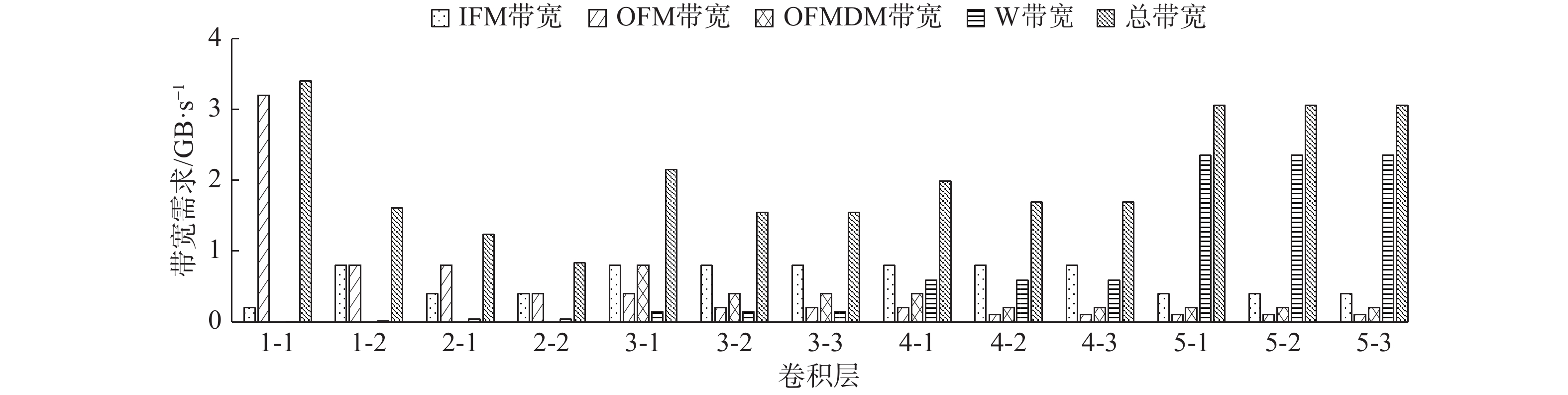Download: 图 14 VGG-16网络极限情况下系统带宽需求 Fig. 14 Limit case system bandwidth requirements of VGG-16 network

4.3 计算时间与有效激活输入密度关系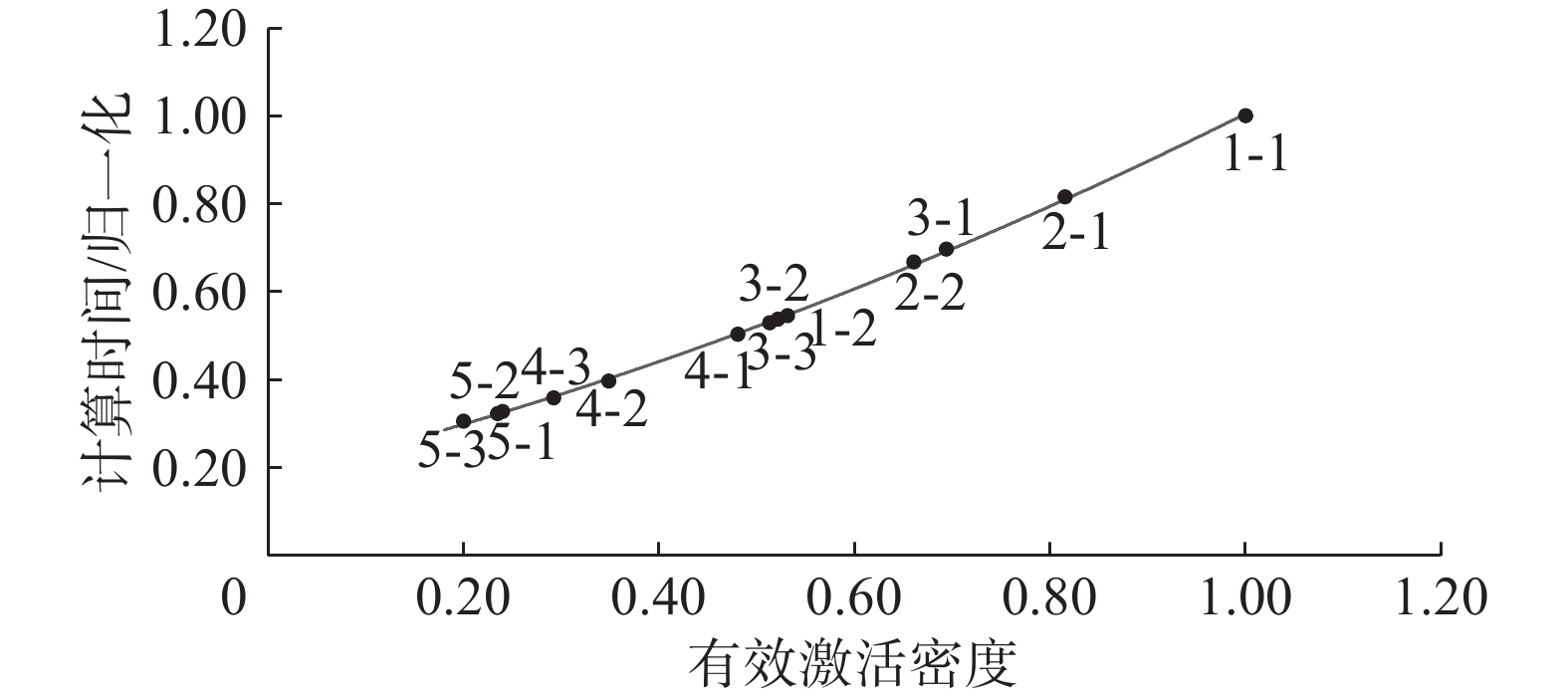Download: 图 15 VGG-16网络计算时间和有效激活输入密度关系 Fig. 15 Relationship between computing time and effective activation input density of VGG-16 network
4.4 卷积神经网络加速器性能对比表 3 稀疏卷积神经网络加速器VGG-16各层计算性能 Tab.3 Sparse convolutional neural network accelerator VGG-16 calculation performance of each layer表 4 与其他卷积神经网络加速器的VGG-16运行性能的比较 Tab.4 Comparison of VGG-16 performance with other convolution neural network accelerators
5 结束语

  RUSSAKOVSKY O, DENG Jia, SU Hao, et al. ImageNet large scale visual recognition challenge[J]. International journal of computer vision, 2014, 115(3): 211-252. (0)  SIMONYAN K, ZISSERMAN A. Very deep convolutional networks for large-scale image recognition[C]// The 3rd International Conference on Learning Representations (ICLR2015).San Diego, CA, 2015. (0)  ZHANG Chen, LI Peng, SUN Guangyu, et al. Optimizing FPGA-based accelerator design for deep convolutional neural networks[C]//Proceedings of 2015 ACM/SIGDA International Symposium on Field-programmable Gate Arrays. New York, NY, USA, 2015. (0)  NATALE G, BACIS M, SANTAMBROGIO M D. On how to design dataflow FPGA-based accelerators for convolutional neural networks[C]//2017 IEEE Computer Society Annual Symposium on VLSI (ISVLSI). Bochum, Germany, 2017. (0)  SHEN Yongming, FERDMAN M, MILDER P. Maximizing CNN accelerator efficiency through resource partitioning[C]//2017 ACM/IEEE 44th Annual International Symposium on Computer Architecture (ISCA). Toronto, ON, Canada, 2016. (0)  MA Yufei, CAO Yu, VRUDHULA S, et al. Optimizing loop operation and dataflow in FPGA acceleration of deep convolutional neural networks[C]//ACM/SIGDA International Symposium on Field-programmable Gate Arrays. Monterey, California, USA, 2017. (0)  SHI Shaohuai, CHU Xiaowen. Speeding up convolutional neural networks by exploiting the sparsity of rectifier units[J]. Computer vision and pattern recognition, 2017, 4: 1-7. (0)  CHEN Y H, KRISHNA T, EMER J S, et al. Eyeriss: an energy-efficient reconfigurable accelerator for deep convolutional neural networks[J]. IEEE journal of solid-state circuits, 2017, 52(1): 127-138. DOI:10.1109/JSSC.2016.2616357 (0)  ALBERICIO J, JUDD P, HETHERINGTON T, et al. Cnvlutin: ineffectual-neuron-free deep neural network computing[C]//2016 ACM/IEEE 43rd Annual International Symposium on Computer Architecture (ISCA). Seoul, South Korea, 2016. (0)  ZHANG Shijin, DU Zidong, ZHANG Lei, et al. Cambricon-X: an accelerator for sparse neural networks[C]//201649th Annual IEEE/ACM International Symposium on Microarchitecture (MICRO). Taipei, China, 2016: 1−12. (0)  HAN Song, LIU Xingyu, MAO Huizi, et al. EIE: efficient inference engine on compressed deep neural network[J]. International symposium on computer architecture, 2016, 44(3): 243-254. DOI:10.1145/3007787.3001163 (0)  Parashar A , RHU M , Mukkara A , et al. SCNN: An accelerator for compressed-sparse convolutional neural networks[C]//The 44th Annual International Symposium. TorontoCanada, 2017. (0)  OLSHAUSEN B A, FIELD D J. Sparse coding with an overcomplete basis set: a strategy employed by V1?[J]. Vision research, 1997, 37(23): 3311-3325. DOI:10.1016/S0042-6989(97)00169-7 (0)  DAYAN P, ABBOTT L F. Theoretical neuroscience: computational and mathematical modeling of neural systems[M]. Cambridge, USA: The MIT Press, 2001. (0)  NAIR V, HINTON G E. Rectified linear units improve restricted Boltzmann machines[C]//Proceedings of the 27th International Conference on International Conference on Machine Learning. Haifa, Israel, 2010. (0)  KRIZHEVSKY A, SUTSKEVER I, HINTON G E. ImageNet classification with deep convolutional neural networks[C]//Proceedings of the 25th International Conference on Neural Information Processing Systems. Lake Tahoe, Nevada, 2012. (0)  HAN Song, POOL J, TRAN J, et al. Learning both weights and connections for efficient neural networks[C]//Proceedings of the 28th International Conference on Neural Information Processing Systems. Montreal, Canada, 2015: 1135−1143. (0)  HAN Song, MAO Huizi, DALLY W J, et al. Deep compression: compressing deep neural networks with pruning, trained quantization and Huffman coding[C]//International Conference on Learning Representations, San Juan, Puerto Rico,2016. (0)  MA Yufei, SUDA N, CAO Yu, et al. Scalable and modularized RTL compilation of convolutional neural networks onto FPGA[C]//201626th International Conference on Field Programmable Logic and Applications (FPL). Lausanne, Switzerland, 2016: 1−8. (0)  KU NG. Why systolic architectures[J]. IEEE Computer, 1982, 15(1): 300-309. (0)  GYSEL P, MOTAMEDI M, GHIASI S. Hardware-oriented approximation of convolutional neural networks[J]. Computer Vision and Pattern Recognition, 2016, 10: 1-8. (0)  DORRANCE R, REN Fengbo, MARKOVIĆ D, et al. A scalable sparse matrix-vector multiplication kernel for energy-efficient sparse-blas on FPGAs[C]//ACM/SIGDA International Symposium on Field-Programmable Gate Arrays. Monterey, California, USA, 2014: 161−170. (0)  QIU Jiantao, WANG Jie, YAO Song, et al. Going deeper with embedded FPGA platform for convolutional neural network[C]//Proceedings of the 2016 ACM/SIGDA International Symposium on Field-Programmable Gate Arrays. Monterey, California, USA, 2016: 26−35. (0)  SUDA N, CHANDRA V, DASIKA G, et al. Throughput-optimized openCL-based FPGA accelerator for large-scale convolutional neural networks[C]//Proceedings of the 2016 ACM/SIGDA International Symposium on Field-Programmable Gate Arrays. Monterey, California, USA, 2016. (0)  ZHANG Chen, FANG Zhenman, ZHOU Peipei, et al. Caffeine: towards uniformed representation and acceleration for deep convolutional neural networks[C]//Proceedings of the 35th International Conference on Computer-Aided Design. Austin, Texas, USA, 2016. (0)